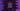# C program to convert meter to yard# C meter to yard conversion program :

Length conversion is a common problem in many applications. You application is getting the value in one unit from the server and you need to convert it before showing it to the user. Or your application takes the input in one unit and convert it to another unit.

In this tutorial, I will show you how to convert one value in meter into yard using C programming language. I will write one simple program, that will take the meter value as input, convert it to yard and print it to the user.

## Meter to yard conversion formula :

``1 meter = 1.09361 yard``

So, for n meters, we need to multiply it with 1.09361 to get the yard value.

### C program :

``````#include <stdio.h>
#define METER_TO_YARD  1.09361

int main()
{
float meter;
float yard;

printf("Enter the value in meter : ");
scanf("%f", &meter);

yard = meter * METER_TO_YARD;

printf("%f meter : %f yard\n",meter,yard);
}``````

### Sample output :

``````Enter the value in meter : 35
35.000000 meter : 38.276348 yard

Enter the value in meter : 45
45.000000 meter : 49.212448 yard``````